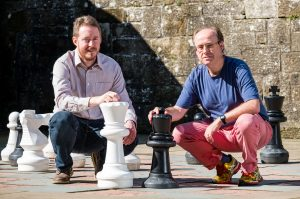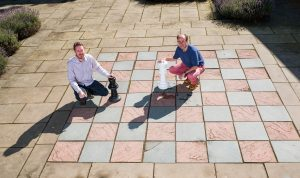# n-Queens Completion is NP-Complete

### Update, 2021

Over the years since we published this research, many people have approached us having solved the n queens puzzle, either for one n like 8 or 1000, or having written an algorithm to solve it for different sizes.  Unfortunately this is not a major result in Computer Science and does not make one eligible to claim the \$1M Clay prize. Many have been disappointed by this so we want to clarify why  this is the case.

It is true that work on this problem could potentially result in the award but only if some exceptionally difficult conditions are met.

• EITHER prove mathematically that NO possible algorithm could solve the n queens completion problem in polynomial time;
• OR prove that there is an algorithm which is guaranteed to solve every instance of the n queens completion problem in polynomial time. Note that in this case the algorithm has to work on the completion version of the problem studied in our paper, not placing queens on an empty board; the algorithm has to give the correct answer on every possible instance given to it; and there has to be a mathematical proof that the algorithm’s runtime is bounded above by some polynomial in the size of the board.  However fast a given algorithm runs when tested, this is not sufficient because there are an infinite number of possible tests available, so a mathematical proof is required.
• AND in either case, prove this at a level that is published in a respected academic source and is widely accepted by research experts as correct.

We are delighted that our work has led so many people to be interested in the problem of solving problems like the n queens puzzle that fascinates us.  But we also apologise for any impression we gave, unintentionally, that a solution to the n queens puzzle could lead to the award of the prize except under the extremely strenuous conditions listed above.

Ian Gent, 10 May 2021

Original Post from 2017:

Ian Gent, Christopher Jefferson and Peter Nightingale have shown that a classic chess puzzle is NP-Complete. Their paper “Complexity of n-Queens Completion” was published in the Journal of Artificial Intelligence Research on August 30, 2017.

The n-Queens puzzle is a classic chess problem: given a chessboard of size n by n, can you place n queens so that no two queens attack each other?  That is, can you place the queens with no two queens are on the same row, column, or diagonal? The n-Queens puzzle has long been known to be simple to solve:  you can solve the problem for all n except 2 and 3, and solutions for all other n can be described in a few lines.  This very simplicity has led to repeatedPeter Nightingale and Ian Gent at Falkland Palace, Wednesday, 17 August 2017.

controversy in Artificial Intelligence (AI). The n-Queens puzzle is often used as a benchmark problem, but good results on the problem can always be challenged because the problem is so simple to solve without using AI methods.

The new work follows a challenge on Facebook on New Year’s Day, 2015, when a friend of Ian’s asked him how hard n-Queens is if some queens were already placed on the board.  It turns out, this version (dating from 1850) of the puzzle is only two years younger than the more famous n-Queens problem. The picture shows Peter (left) and Ian (right) with queens on the board at positions suggested by Nauck in 1850, the squares b4 and d5.  Can you put another 6 queens on the board so that the entire board is a solution of 8-Queens?  The general version with some number of queens preplaced on an n by n board is the n-Queens Completion puzzle.With queens at b4 and d5, can you place 6 more queens to get a solution to the 8-queens puzzle? ©Stuart Nicol Photography, 2017

Ian, Christopher and Peter have shown that the n-Queens puzzle is in fact hard, not simple.  It belongs to the complexity classes NP-Complete and #P-Complete. Other NP-Complete problems include the “travelling salesperson problem”, finding cliques in graphs, and many other important problems, from scheduling to circuit layout. This puts n-Queens Completion at the centre of the most important theoretical problem in computer science — it has long been known that either all NP-complete problems are easy, or none of them are. Most computer scientists believe that this means there is no efficient algorithm possible for solving this problem, compared to the very simple techniques long known for n-Queens.
The importance of this work is that it provides researchers with a benchmark that can be used for evaluating AI techniques. Moreover, it helps to explain why so many AI techniques have been used on the n-Queens puzzle. Most techniques do most of their work with some queens partially placed, using some form of (hopefully intelligent) trial and error. In fact it turns out that many researchers – in order to solve a simple problem – have solved it by turning the simple problem of n-Queens into the hard problem of n-Queens Completion.
It does seem that AI researchers should not use n-Queens as a benchmark, but the very closely related n-Queens Completion puzzle is a valid benchmark. As well as the theoretical results, the paper shows how example instances can be generated which appear to be hard in practice. Some caution is still needed, though. It does seem to be quite hard to generate hard instances of n-Queens Completion.
The University has also issued an article on the same paper, under the title “Simple” chess puzzle holds key to \$1m prize